# A low pass FIR filter for ECG Denoising in VHDL

## In this VHDL project, a simple low pass FIR filter is implemented in VHDL for ECG Denoising. VHDL code for the FIR filter is fully presented.

#### The VHDL code for the FIR filter is simulated and verified by comparing the simulated results in Modelsim with the correct results generated from Matlab. Sample ECG inputs are provided in input.txt files, the VHDL filter code reads those ECG files, apply digital filtering, and write the results into output.txt files for verification.It is obvious that one of the most critical steps in ECG digital signal processing is noise filtering because ECG signals are noisily affected by many different sources such as Baseline Wander, EMG interference, and power line noise. Those noises can be reduced by many filters as shown in the figure above. This project is to implement a low pass FIR Filter in VHDL to reduce high-frequency noise and power-line interference.

The specification of the filter as follows:

• Order: 10
• Tap: 11
• 8-bit signed integer coefficients as shown below:#### In this project, the regular implementation of the FIR filter as shown in the following figure is implemented in VHDL.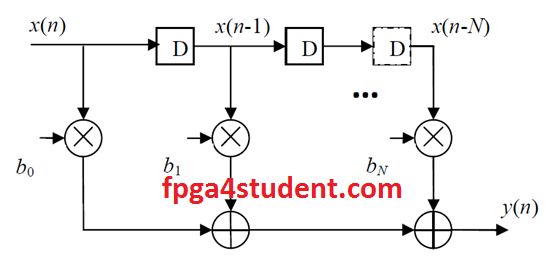### VHDL code for the low pass FIR filter:

```Library IEEE;
USE IEEE.Std_logic_1164.all;
USE IEEE.Std_logic_signed.all;
-- fpga4student.com: FPGA projects, VHDL projects, Verilog projects
-- LOW pass FIR filter for ECG Denoising
-- VHDL project: VHDL code for FIR filter
entity FIR_RI is  -- VHDL projects
generic (
input_width          : integer     :=8               ;-- set input width by user
output_width     : integer     :=16               ;-- set output width by user
coef_width          : integer     :=8               ;-- set coefficient width by user
tap                    : integer     :=11               ;-- set filter order
guard               : integer     :=0)               ;-- log2(tap)+1
port(
Din          : in      std_logic_vector(input_width-1 downto 0)     ;-- input data
Clk          : in      std_logic                                             ;-- input clk
reset     : in      std_logic                                             ;-- input reset
Dout     : out      std_logic_vector(output_width-1 downto 0))     ;-- output data
end FIR_RI;
architecture behaivioral of FIR_RI is
-- N bit Register
component N_bit_Reg
generic (
input_width          : integer     :=8
);
port(
Q : out std_logic_vector(input_width-1 downto 0);
Clk :in std_logic;
reset :in std_logic;
D :in std_logic_vector(input_width-1 downto 0)
);
end component;
-- fpga4student.com: FPGA projects, VHDL projects, Verilog projects
type Coeficient_type is array (1 to tap) of std_logic_vector(coef_width-1 downto 0);
-----------------------------------FIR filter coefficients----------------------------------------------------------------
constant coeficient: coeficient_type :=
(     X"F1",
X"F3",
X"07",
X"26",
X"42",
X"4E",
X"42",
X"26",
X"07",
X"F3",
X"F1"
);
----------------------------------------------------------------------------------------------
type shift_reg_type is array (0 to tap-1) of std_logic_vector(input_width-1 downto 0);
signal shift_reg : shift_reg_type;
type mult_type is array (0 to tap-1) of std_logic_vector(input_width+coef_width-1 downto 0);
signal mult : mult_type;
type ADD_type is array (0 to tap-1) of std_logic_vector(input_width+coef_width-1 downto 0);
begin
-- fpga4student.com: FPGA projects, VHDL projects, Verilog projects
shift_reg(0)     <= Din;
mult(0)<= Din*coeficient(1);
GEN_FIR:
for i in 0 to tap-2 generate
begin
-- N-bit reg unit
N_bit_Reg_unit : N_bit_Reg generic map (input_width => 8)
port map ( Clk => Clk,
reset => reset,
D => shift_reg(i),
Q => shift_reg(i+1)
);
-- filter multiplication
mult(i+1)<= shift_reg(i+1)*coeficient(i+2);
-- filter conbinational addition
end generate GEN_FIR;
end Architecture;

Library IEEE;
USE IEEE.Std_logic_1164.all;

-- fpga4student.com: FPGA projects, VHDL projects, Verilog projects
-- LOW pass FIR filter for ECG Denoising
-- VHDL project: VHDL code for FIR filter
-- N-bit Register in VHDL
entity N_bit_Reg is
generic (
input_width          : integer     :=8
);
port(
Q : out std_logic_vector(input_width-1 downto 0);
Clk :in std_logic;
reset :in std_logic;
D :in std_logic_vector(input_width-1 downto 0)
);
end N_bit_Reg;
-- fpga4student.com: FPGA projects, VHDL projects, Verilog projects
architecture Behavioral of N_bit_Reg is
begin
process(Clk,reset)
begin
if (reset = '1') then
Q <= (others => '0');
elsif ( rising_edge(Clk) ) then
Q <= D;
end if;
end process;
end Behavioral;
```

### Testbench VHDL code for the FIR filter:

``` Library IEEE;
USE IEEE.Std_logic_1164.all;
USE IEEE.numeric_std.all;
Use STD.TEXTIO.all;
-- fpga4student.com: FPGA projects, VHDL projects, Verilog projects
-- VHDL project: VHDL code for FIR filter
-- Testbench VHDL code for FIR Filter
entity TB_FIR is
end TB_FIR;
architecture behaivioral of TB_FIR is
Component FIR_RI is
generic (
input_width          : integer     :=8               ; -- set input width by user
output_width     : integer     :=16               ; -- set output width by user
coef_width          : integer     :=8               ; -- set coefficient width by user
tap                    : integer     :=11               ; -- set filter order
guard               : integer     :=4)                    ; -- log2(tap)+1
port(
Din          : in      std_logic_vector(input_width-1 downto 0)     ;     -- input data
Clk          : in      std_logic                              ;               -- input clk
reset     : in      std_logic                                   ;               -- input reset
Dout     : out      std_logic_vector(output_width-1 downto 0))     ;-- output data
end Component;
signal Din          :      std_logic_vector(7 downto 0)     ;
signal Clk          :      std_logic:='0'                              ;
signal reset     :      std_logic:='1'                              ;
signal output_ready     :      std_logic:='0';
signal Dout          :      std_logic_vector(15 downto 0)     ;
signal input: std_logic_vector(7 downto 0);
file my_input : TEXT open READ_MODE is "input101.txt";
file my_output : TEXT open WRITE_MODE is "output101_functional_sim.txt";
begin
-- fpga4student.com: FPGA projects, VHDL projects, Verilog projects
FIR_int : FIR_RI
generic map(
input_width          =>     8,
output_width     =>     16,
coef_width          =>     8,
tap                 =>     11,
guard               =>     0)
port map     (
Din                    => Din,
Clk                    => Clk,
reset               => reset,
Dout               => Dout
);
process(clk)
begin
Clk          <= not Clk after 10 ns;
end process;
reset     <= '1', '1' after 100 ns, '0' after 503 ns;
-- fpga4student.com: FPGA projects, VHDL projects, Verilog projects
-- Writing output result to output file
process(clk)
variable my_input_line : LINE;
variable input1: integer;
begin
if reset ='1' then
Din <= (others=> '0');
input <= (others=> '0');
elsif rising_edge(clk) then
Din <= std_logic_vector(to_signed(input1, 8));
--Din<=input(7 downto 0);
end if;
end process;
process(clk)
variable my_output_line : LINE;
variable input1: integer;
begin
if falling_edge(clk) then
if output_ready ='1' then
write(my_output_line, to_integer(signed(Dout)));
writeline(my_output,my_output_line);
end if;
end if;
end process;

end Architecture;
```
After running simulations in Modelsim, the filtered output is written into output.txt for verification. The verification is done by comparing the output file with the correct result generated from Matlab.
Download sample inputs and output from Matlab

1.Like to run this on my Arty board but is having a hard time to get the ADC configured.Any help for a ADC interface available?

1.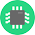Find an ADC chip or module such as MCP3001 or PMOD ADC Digilent

2.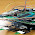what is sampling frequency?

1.This is a regular implementation of FIR Filter. It is not designed by using frequency sampling method.

3.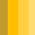Hey,
I've got a problem with this line in the code : write(my_output_line, to_integer(signed(Dout))); The compilator in ISE is saying : "Second argument of write must have a constant value". I dont' know how to fix this issue. Thanks for your help

1.You compiled or synthesized the testbench, that's why you got that error. You only run simulation with the testbench. Synthesize the top level only (FIR_RI).

2.Thanks for your quick help. Yes indeed, I've compiled the test bench. So, the testbench just has to be written as a module, I thought it was the main module (top level) to compile ? Sorry, I'm a beginner !!

4.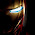Thank you for the code, i am having a problem with input output and coefficient width . what are they for kaiser window or how to calculate them??

1.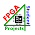They are defined by users

5.Hi Loi,
Mình gặp lỗi này khi chạy simulation."File input101.txt could not be opened"
Mình đã download file input về nhưng chưa biết phải đặt vào thư mục nào để chương trình đọc được
Mình cần phải đặt vào đâu nhỉ?
Cảm ơn bạn!

1.Bo vao folder ban chay simulation nhe.

6.Does anyone have a MATLAB program with this filter implemented that gives the same output? I tried creating FIR filter using coefficients from this project, but I am getting completely different output.

1.if you use this VHDL code, you will generate the same output as Matlab.

7.will it work on xilinx ise too?

1.It was simulated in Xilinx ISE.

8.hi van. thank you for code.

i have a doubt that you have applied text input through text bench, then how to apply it for fpga.

1.In that case, you need to create a synthesizable model to pass the text file data to the FIR filter.

9.Will it work for Altera Quartus Prime? How do I get the input file?

1.sure it works. The input files are given above.

10.Cho em hỏi, em copy code vào file .vht, rồi start compilation thì không có lỗi gì. Nhưng khi vào folder chạy simulation (đã có file input trong đó) thì không thấy file output đuôi .txt đâu. Không biết đây là lỗi gì hay cách em chạy mô phỏng bị sai? Mong được giúp đỡ. Em dùng Quartus II 12.1.

1.You have to run simulation until output_ready = '1' so that the output file is written.

11.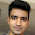Sample input and output files are not there anymore in the link you have provided. I request you to kindly provide it again.

1.Sorry I have updated the link.

12.I did run modelsim simulator keeping 'input101.txt' file in project folder. i got 'output101_functional_sim.txt' file which is not same as 'output101.txt' sample file provided by you in the link.
Number of values in 'output101_functional_sim.txt' file is very less than the number of values in 'output101.txt' file. Please help regarding it.

1.Double check your simulation. The code was verified and all the outputs were approximately matched with the provided samples.